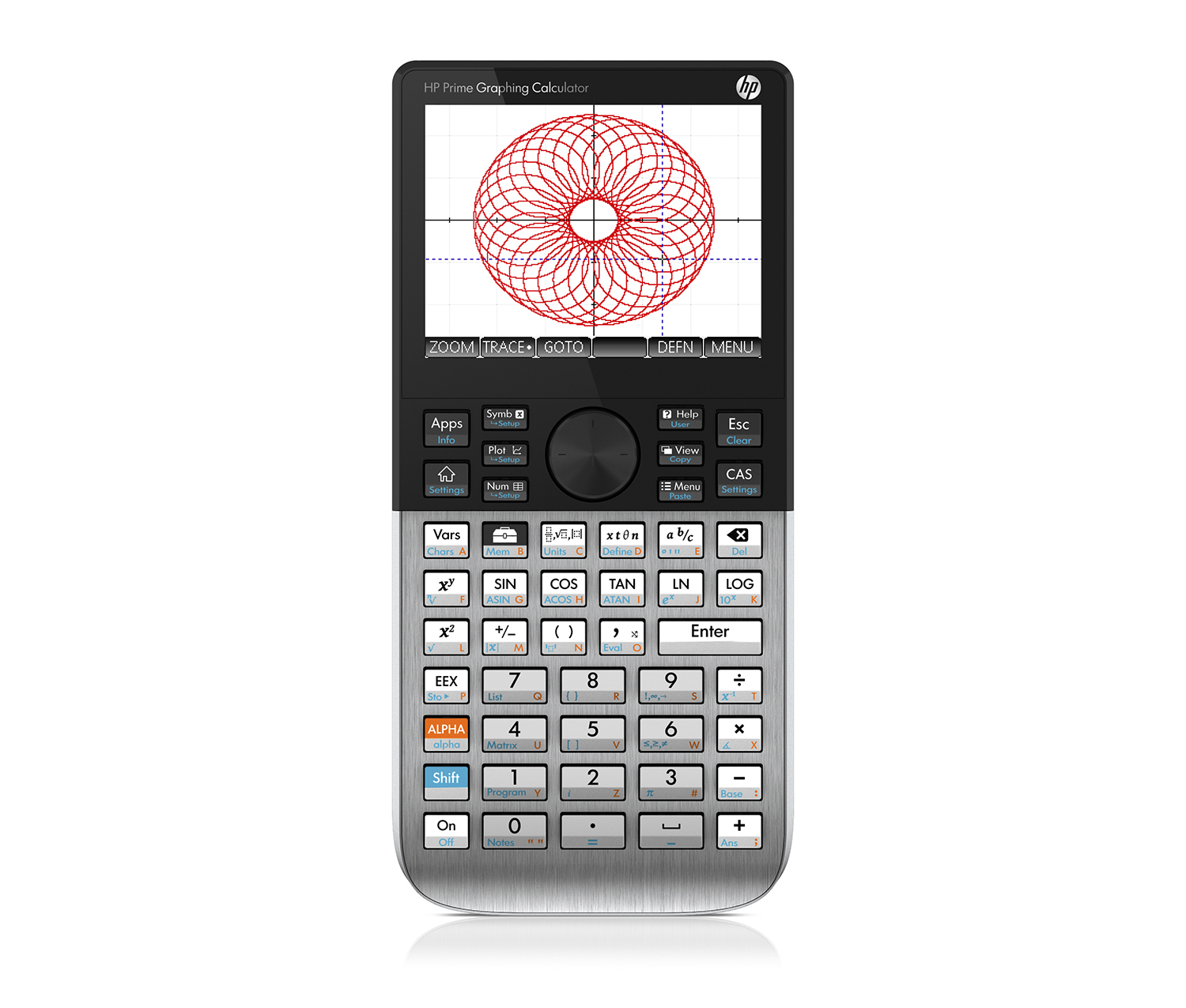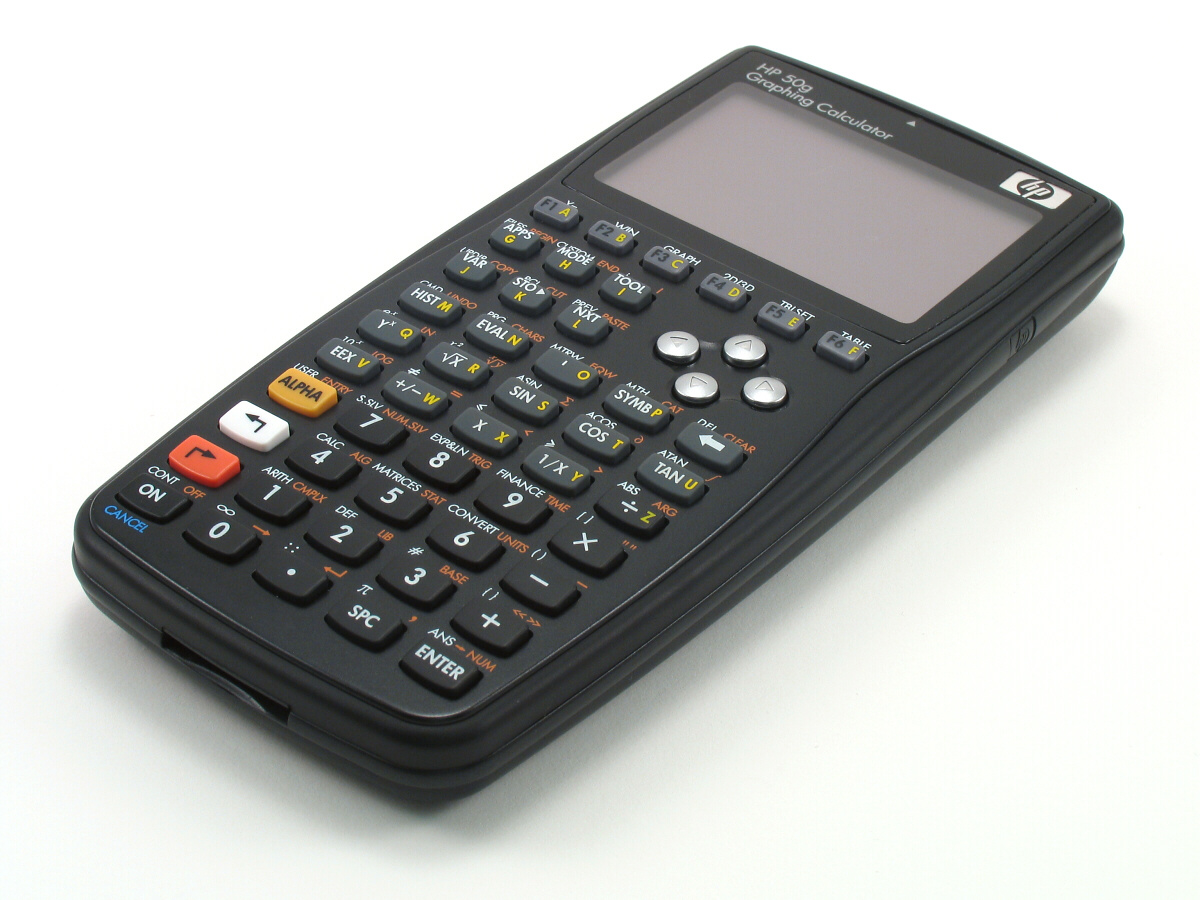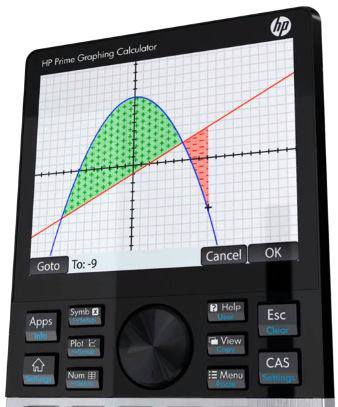## Mathematics: the Queen of the SciencesGauss referred to mathematics as "the Queen of the Sciences". In the original Latin Regina Scientiarum, as well as in German Königin der Wissenschaften, the word corresponding to science means a "field of knowledge", and this was the original meaning of "science" in English, also; mathematics is in this sense a field of knowledge. The specialization restricting the meaning of "science" to natural science follows the rise of Baconian science, which contrasted "natural science" to scholasticism, the Aristotelean method of inquiring from first principles. The role of empirical experimentation and observation is negligible in mathematics, compared to natural sciences such as psychology, biology, or physics. Albert Einstein stated that "as far as the laws of mathematics refer to reality, they are not certain; and as far as they are certain, they do not refer to reality." More recently, Marcus du Sautoy has called mathematics "the Queen of Science ... the main driving force behind scientific discovery".

Calculus is the mathematical study of change, in the same way that geometry is the study of shape and algebra is the study of operations and their application to solving equations. It has two major branches, differential calculus (concerning rates of change and slopes of curves), and integral calculus (concerning accumulation of quantities and the areas under and between curves); these two branches are related to each other by the fundamental theorem of calculus. Both branches make use of the fundamental notions of convergence of infinite sequences and infinite series to a well-defined limit. Generally, modern calculus is considered to have been developed in the 17th century by Isaac Newton and Gottfried Leibniz. Today, calculus has widespread uses in science, engineering and economics and can solve many problems that algebra alone cannot. Modern calculus was developed in 17th century Europe by Isaac Newton and Gottfried Wilhelm Leibniz, but elements of it have appeared in ancient Greece, China, medieval Europe, India, and the Middle East.## Hewlett-Packard RPN Graphing Calculators